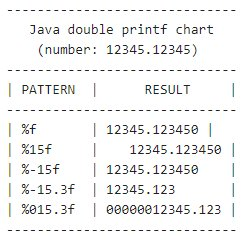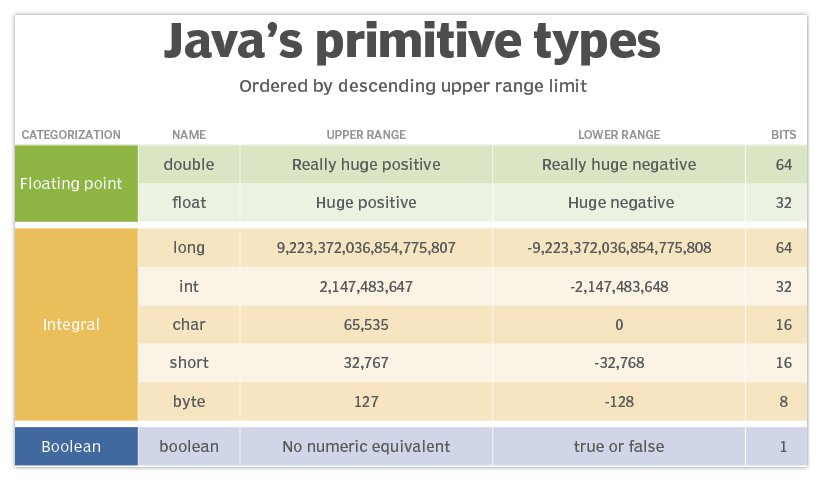# Java double decimal precision

## Java double precision

The precision of a double in Java is 10-324 decimal places, although true mathematical precision can suffer due to issues with binary arithmetic. To simplify display, you can use %d printf notation to format a Java double’s precision to two decimal places, while internally the double variable’s precision is maintained.

## What is a double’s range of precision?

The Double wrapper class provides two properties that represent the range of a Java double:

• MIN_VALUE
• MAX_VALUE

These properties can be referenced in code as follows:

```System.out.println(Double.MAX_VALUE);
System.out.println(Double.MIN_VALUE);```

Execution of the code above provides the following information about the range and precision of a double in Java:

1. The lower range of a double is 4.9 E-32
2. The upper range of a double is 1.7976931348623157 E308

The exact range can vary depending on how the JVM is implemented on a given operating system or hardware architecture.

## How to set double precision to 2 decimal places?

A double in Java is a 64-bit number, and the 64-bit precision of a double never changes within a Java program.

However, to format the output of a double to two decimal places, simply use the printf method and %.2f as the specifier.

```public class JavaDoublePrecision {
/* Print Java double to 2 decimals of precision. */
public static void main(String[] args) {
double big   = 1234.12345;
float  small = 1234.12345f;
System.out.printf("%,.2f :: %,.3f", big, small);
/* Example prints: 1,234.12 :: 1234.123 */
}
}```

The logic of the above code where we output a Java double to two decimals of precision works as follows:

• The `%f` specifier represents a double or float value to format.
• The decimal and number after the `%` specify the number of decimals to print.
• A comma after the `%` sign adds a grouping separator for large numbers.You can use printf and the %f specifier to format a double to two decimal places of precision.

## Loss of Java double precision in math

Binary numbers don’t always map cleanly to the base-10 number system.

As a result, there can sometimes be a loss of precision in even the simplest of operation.

In the following example, the addition of 5.6 and 5.8 results in a value of 11.3999, not 11.4

```double sum = 0;
sum = sum + 5.6;
sum = sum + 5.8;
System.out.println(precision); // prints 11.399999999999999```

For the most precise double calculations in Java, the JDK provides a special class named BigDecimal.

For any calculations that require extreme precision, use this class instead.Java’s float and double data types are the only two floating point primitive types in the API.

• How to test a predictive model

Strategies for testing predictive models and analytics emphasize data quality, real-time testing and code redundancy, as well as ...

• The dos and don'ts of visual testing

The visual aspect of an application is an important part of UX. Defects can potentially result in lost sales and damaged ...

• 3 QA testing tools to consider

QA testers need to be able to put applications and APIs through their paces. Here are some examples of tools that can help with ...

Close